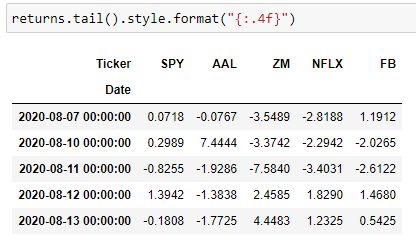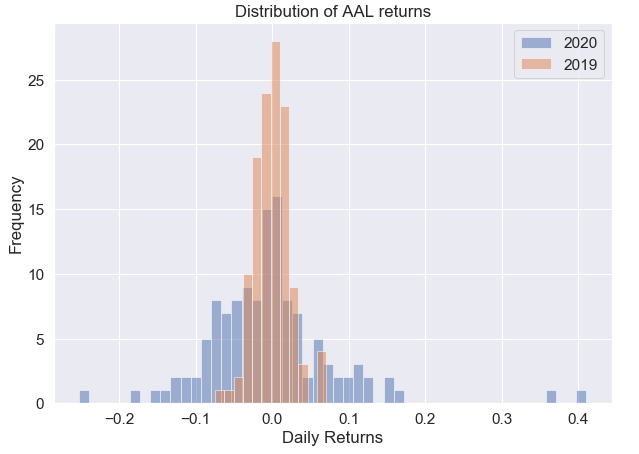python初学者

# 项目背景 (Project Background)

Besides working to earn an income, investing is the best way to reach your financial goals in life, be it saving for a new car, starting a new business, or ensuring a comfortable retirement. The idea of passively increasing the value of my assets over time without doing anything (besides buying stocks and holding it) intrigued me as I pursued financial independence. However, I didn’t know where to start. How do I make a decision on what stocks to buy? How can I understand company’s performance by looking at the stock market? Can I pull historical stock market data to create buy/sell signals and automate alerts or even trades?

Without getting too deep into the financial background of each tool, I will show you how I use Python to pull historical stock data, visualize growth and decay trends, understand returns, estimate risks using returns standard deviations, and identify undervalued stocks to buy.

1. Getting Stock Market Data/Data Preparation

获取股票市场数据/数据准备
2. Exploratory Data Analysis

探索性数据分析
3. Visualizing Prices over Time

随时间可视化价格
4. Conducting Risk and Returns Analysis

进行风险与收益分析
5. Seeing Trends with Simple Moving Averages

通过简单的移动平均线查看趋势
6. Finding Under/Over-value Stocks with Bollinger Band Plots

使用布林带图查找低/高价值股票

In other words, this article will show you the skeleton “how the analysis is done” using Python, but the “interpretation” meat of the analysis will be discounted. For the interested reader, I dig deeper into real-world examples and interpretation in another 6-part series. The Python code is the exact same for the 6-part series as covered in this blog, but there is more context given to the analysis there. I highly encourage you to read that series if you have the time. For now, I’ll cover the distilled, Python-focused work in this article. Let’s begin!

# 获取数据 (Getting the Data)

There are multiple ways to pull stock market data, each with their own advantages. Here, I will use the pandas-datareader package. Make sure you have this installed if you plan on following along with the coding. Using the pandas-datareader package, we’ll use Yahoo Finance as our source of information. All you need to provide is the start and end dates of your analysis period , as well as a list of ticker symbols of companies that you’re interested in.

# Set the start and end dates for your analysis. The end date is set to current day by defaultstart_date = '2018-01-01'end_date = datetime.date.today().strftime('%Y-%m-%d')# List the ticker symbols you are interested in (as strings) tickers = ["SPY", "AAL", "ZM", "NFLX", "FB"]# I want to store each df separately to do Bollinger band plots later oneach_df = {}for ticker in tickers:    each_df[ticker] = data.DataReader(ticker, 'yahoo', start_date, end_date)# Concatenate dataframes for each ticker together to create a single dataframe called stocksstocks = pd.concat(each_df, axis=1, keys = tickers)# Set names for the multi-index stocks.columns.names = ['Ticker Symbol','Stock Info']

The stocks dataframe should look something like this.

# 探索性数据分析 (Exploratory Data Analysis)

In this step, I try to better understand what I am looking at. I try to discover patterns, detect anomalies, and answer questions like How many data points do I have? Does anything look strange? Am I missing any information?

There are a few standard operations I do when looking at any new data set. These include looking at the pandas.DataFrame.shape attribute, the .info() method to see the columns and their datatypes, the .describe() method to understand the descriptive statistics of the numerical data, and the .isnull() method to see missing data.

Here is the output of stocks.shape:

Here is the output of stocks.info():

Here is the output of stocks.describe():

Here is the output of stock.isnull() visualized in a heatmap:

# 随时间可视化数据 (Visualization of Data over Time)

First, let’s create a dataframe called ‘closing_prices_df’ with only the adjusted closing prices of each stock. We can do this easily using the cross section method of pandas.DataFrame.xs().

# Create a dataframe for the closing_prices of the stocksclosing_prices_df = stocks.xs(key='Adj Close',axis=1,level=1)

Then, we can use the plotly package to plot closing prices over time.

# Create a dataframe for the closing_prices of the stocksclosing_prices_df = stocks.xs(key='Adj Close',axis=1,level=1)# Using plotly to create line plot of stock closing prices over time. # You can double click on the ticker symbols in the legend to isolate each tickerfig = px.line(closing_prices_df, x=closing_prices_df.index, y=closing_prices_df.columns, title=”Adjusted Closing Prices”)fig.update_layout(hovermode=’x’,yaxis_title=”Price”)fig.show()

Besides looking at closing prices over time, it is helpful to summarize much more information within candlestick plots. Candlestick charts are a commonly used financial visual to show price movements in securities. They are densely packed with information, including single day open, close, high, and low prices.

Here, we create our own candlestick chart for our companies of interest using plotly.

import plotly.graph_objects as go# User input ticker of interestticker = "NFLX"fig = go.Figure(data=[go.Candlestick(x=each_df[ticker].index,                open=each_df[ticker]['Open'],                high=each_df[ticker]['High'],                low=each_df[ticker]['Low'],                close=each_df[ticker]['Close'])])fig.update_layout(    title='Candlestick Chart for ' + ticker,    yaxis_title='Price',    xaxis_title='Date',    hovermode='x')fig.show()

Here is what an example of what the candlestick plot looks like.

Again, candlestick charts are packed dense with information, so there are many traders who build trading strategies around them. I discuss them a little more in my other post here.

# 风险与收益分析 (Risk and Returns Analysis)

To make well-informed trades, it is important to understand the risk and returns of each stock. First, let’s create a new dataframe to store the daily percent changes in closing prices. I call this the ‘returns’ dataframe.

# Create a new df called returns that calculates the return after each day.returns = pd.DataFrame()# We can use pandas pct_change() method on the 'Adj Close' column to create a column representing this return value. for ticker in tickers:    returns[ticker]=closing_prices_df[ticker].pct_change()*100Figure 8: Tail of the returns dataframe to look at daily returns

Using the dataframe in Figure 8, we can now see how much gain/loss a stock experienced day by day. However, it’s difficult to see the big picture when looking at all these numbers.

Let’s summarize the data and look at the average daily returns. We can do this simply by using the .mean() function.

In Figure 9, we are looking at the average returns over ~2.5 years. In other words, if you invested in Facebook (FB) 2.5 years ago, you can expect the value of your investments to have grown 0.08% each day.

I encourage you to play with the returns data as it is quite informational. Some examples of things to look at include best and worst single day returns, which I show in this blog.

Next, let’s take a look at risks. One of the fundamental methods of understanding risk of each stock is through standard deviations on the rate of returns. Let’s calculate the standard deviations of the returns using the code below in Figure 10.

We can break down the standard deviation information using a distribution plot.

# User input ticker of interestticker = “AAL”a = returns[ticker].loc[‘2020–01–03’:’2020–07–01'].dropna()b = returns[ticker].loc[‘2019–01–03’:’2019–07–01'].dropna()plt.figure(figsize = (10,7))a.plot(kind=’hist’, label=’2020', bins=50, alpha=0.5)b.plot(kind=’hist’, label=’2019', bins=12, alpha=0.5)plt.title(‘Distribution of ‘ + ticker + ‘ returns’)plt.xlabel(‘Daily Returns (%)’)plt.legend()plt.show()Figure 11. American Airline’s (AAL) distributions of the daily returns compared 2019–2020

Now that we’ve looked at both risk and returns, let’s pull them together into one graph.

fig = px.scatter(returns, x=returns.mean(), y=returns.std(), text=returns.columns, size_max=60, labels={                     "x": "Daily Expected Returns (%)",                     "y": "Risk",                 },                title="Stock Risk Vs Returns")fig.update_xaxes(zeroline=True, zerolinewidth=2, zerolinecolor='Black')#, range=[-0.005, 0.01])fig.update_yaxes(zeroline=True, zerolinewidth=2, zerolinecolor='Black')#, range=[-0.01, 0.1])fig.update_traces(textposition='top center')fig.show()

# 简单移动平均趋势 (Simple Moving Average Trends)

There are many ways to plot moving averages, but for simplicity, here I use the cufflinks package to do it for me.

# The cufflinks package has useful technical analysis functionality, and we can use .ta_plot(study=’sma’) to create a Simple Moving Averages plot# User input ticker of interestticker = “NFLX”each_df[ticker][‘Adj Close’].ta_plot(study=’sma’,periods=)

The moving average smooths out the day-to-day volatility and better displays the underlying trends in a stock price. The higher the time window for the moving average, the clearer the direction of the trend. However, it is also less sensitive to sudden shifts in market dynamics.

You can do a lot with multiple moving averages and comparing them over time. In my other blog, I talk about two trading strategies called the death cross and golden cross.

# 布林带图 (Bollinger Band Plots)

One of the simplest methods I found to plot Bollinger bands using Python is with the cufflinks package.

# User input ticker of interestticker = "SPY"each_df[ticker]['Close'].ta_plot(study='boll', periods=20,boll_std=2)

As I write in my other post, “One major use case for Bollinger band plots is to help understand undersold vs. oversold stocks. As a stock’s market price moves closer to the upper band, the stock is perceived to be overbought, and as the price moves closer to the lower band, the stock is more oversold.”

In my opinion, this is the most powerful analytical tool discussed in this article. I would definitely spend time understanding Bollinger bands and how to use them. For an introduction, please refer to this.

# 结论 (Conclusion)

And with those few lines of Python code, you should get a jumpstart in Python financial analysis! This blog was meant to showcase the bare-bones code behind my personal financial analysis project. However, as any data scientist knows, domain knowledge is just as critical to understanding data as the coding behind it. If you have time, I highly encourage you to supplement your understanding of this code with financial fundamentals in my 6-part series.

As I’m learning every day, the power of Python is vast and far-reaching. There are a lot of analyses that we can do in Python due to its ability to process large data quickly. What are some things you would be interested to do or see in Python? Let me know in the comments!

python初学者

10-07
04-09229311-302040
08-156506
11-015098
11-2477
04-092890
03-253万+
10-281194
10-28684
06-152803
10-28516
01-1422
10-19
10-19
12-234233点击重新获取扫码支付余额充值